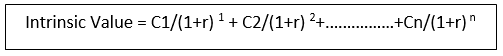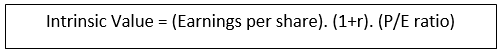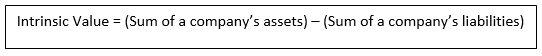# Intrinsic Value

• Updated on

## What is intrinsic value?

Intrinsic value refers to the calculated value of an asset, investment, or a company. The value of an asset or investment can be calculated by financial models or other sophisticated measures. In finance, intrinsic value can be understood as the value that an investor is willing to pay for an investment, given the risk associated with it.

Intrinsic value can be understood as a perceived value and not the actual value. Therefore, it may not always be equal to the current market price of an asset or an investment. It is a measure based only on internal factors, unlike other measures, which rely on relative tools to assess an investment. The most basic measure of intrinsic value is the net present value of an investment.

Intrinsic value is also used in options pricing. The intrinsic value of an option refers to the difference between the market price of an underlying asset and the exercise price of an option or a warrant, but not less than 0.

## What are the factors affecting intrinsic value?

Investors can use fundamental analysis to find the intrinsic value of an investment. This method involves looking at the qualitative as well as the quantitative measures.

Qualitative measures involve the business model, governance, and market factors. These items are specific to the business. Quantitative factors include financial ratios and analysis of the financial statement. These factors depict how well a business does.

There are other perceptual factors which help businesses calculate the relative worth of an asset. These factors can be arrived at through the help of a technical analysis. An asset or a company may be overvalued or undervalued, depending on the strength of the model used to calculate the value.

Both qualitative and quantitative measures are used to calculate the value of an asset.

## How is intrinsic value calculated?

There are various methods of calculating the value of an asset or a company, which are used by financial analysts. These include:

• Discounted Cash Flow Analysis: In this method, an analyst determines the future cash flow attached to an investment and discounts this value to the present day. This method involves the following steps:
1. Forecasting the future cash flows of a company (or investment).
2. Arrive at the present value of each cash flow.
3. Add the present discounted values to find the intrinsic value.

The future cash flows can be arrived at with the help of the previous cash flow statement. The past streams of cash can be a good projection of how a company or an asset would perform in the future.

After the future cash flows are estimated, the subsequent process is not as difficult. The formula for present discounted value can be used to find the intrinsic value. The formula is:In the formula, C1, C2,…Cn refer to the estimates of the future cash flows for periods 1, 2,…n, and r refers to the discount rate or interest rate offered on other investments. A present value analysis is the opposite of a future forecast as it discounts the value obtained in the future down to the interest set up in the current time.

• Financial metric: There can be financial metrics which depict the value of a stock. These include the Price-to-earnings ratio. The formula to use P/E ratio to calculate intrinsic value is:In the formula, r refers to the expected growth rate of earnings. The value obtained from this method would give the intrinsic value of a single share.

• Asset-based valuation: This is a relatively simpler method of valuating the intrinsic value of a company.The negative aspect of using this method is that it does not incorporate any prospects of future growth in a company. Asset-based valuation may also give a value which is lesser than other methods of valuation of a company.

## What are the problems with intrinsic value analysis?

There is no rigid method to which analysts must feel constrained. The valuation of future cash flows might be different from analyst to analyst. The model used to arrive at the intrinsic value can also differ among companies and analysts.

This makes intrinsic valuation a subjective analysis method. The assumptions used to make a present value measurement can vary. The intrinsic value is sensitive to any changes in the model used to determine it.

Most importantly, intrinsic value analysis is only a projection of the future and not an accurate measure. It can’t be used to make future predictions and is to be understood only as an estimate. The differences in valuations obtained by various methods make the method more subjective and drives the measurement away from the real value.

## How is intrinsic value analysis useful?

Intrinsic value helps investors analyse which stocks are trading for lesser than their estimated value. Buying a share which is trading for less than the intrinsic value could prove to be beneficial to an investor.

However, investors must be careful before using intrinsic value as an analysis tool. Certain analysts might undervalue a stock, while others may overvalue it. Each method of measurement must be analysed carefully, before being accepted as a valid tool to decide.

##### Top ASX Listed Companies

Definition:
We use cookies to ensure that we give you the best experience on our website. If you continue to use this site we will assume that you are happy with it. OK